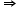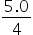Maths-
General
Easy

Question

# Soda is on sale for \$0.20 off each 12-pack. A man bought 4 12-packs and paid a total of \$4.20. How much did each 12-pack originally cost?.Hint:

## The correct answer is: \$1.25.

### Hint: ○ Form the equation according to given information..○ Take variable values as x or any alphabet.○ Solve the equation to get value of x.. Step by step explanation: ○ Given:discount on each 12-pack soda = \$0.20.No of pack brought = 4.total money paid = \$4.20.○ Step 1:○ Let the original price of 12-pack soda be x.∴  purchasing price of one 12- pack soda will be,(original price - discount )(x - 0.20 )Total price paid for 4 12-pack soda will be,(original price - discount )  4(x - 0.20 )  4(4x - 0.8 )○ Step 2:○ As he pays total amount of \$4.20.∴  4.20 = ( 4x - 0.8 )4.2 + 0.8 = 4x5.0 = 4xx =x = 1.25 Final Answer: Hence, the original price of one 12-pack soda is \$1.25.#### With Turito Foundation.#### Get an Expert Advice From Turito.